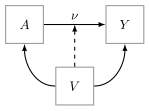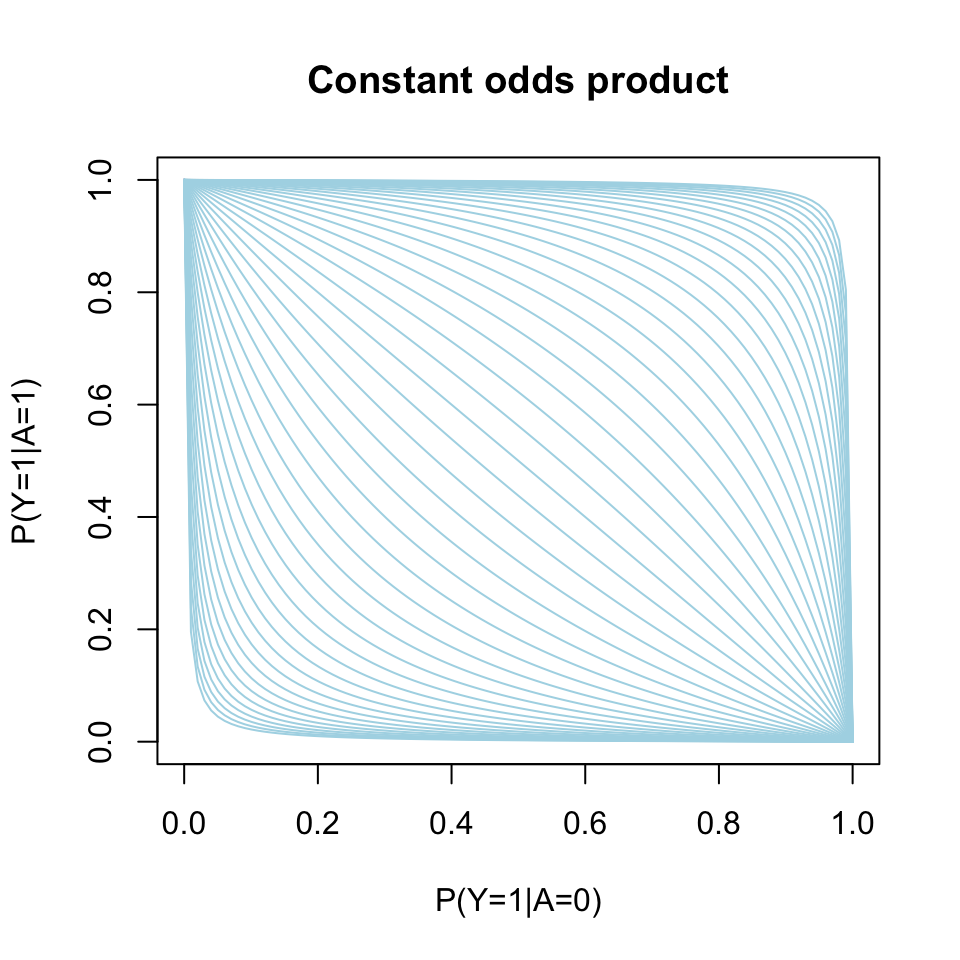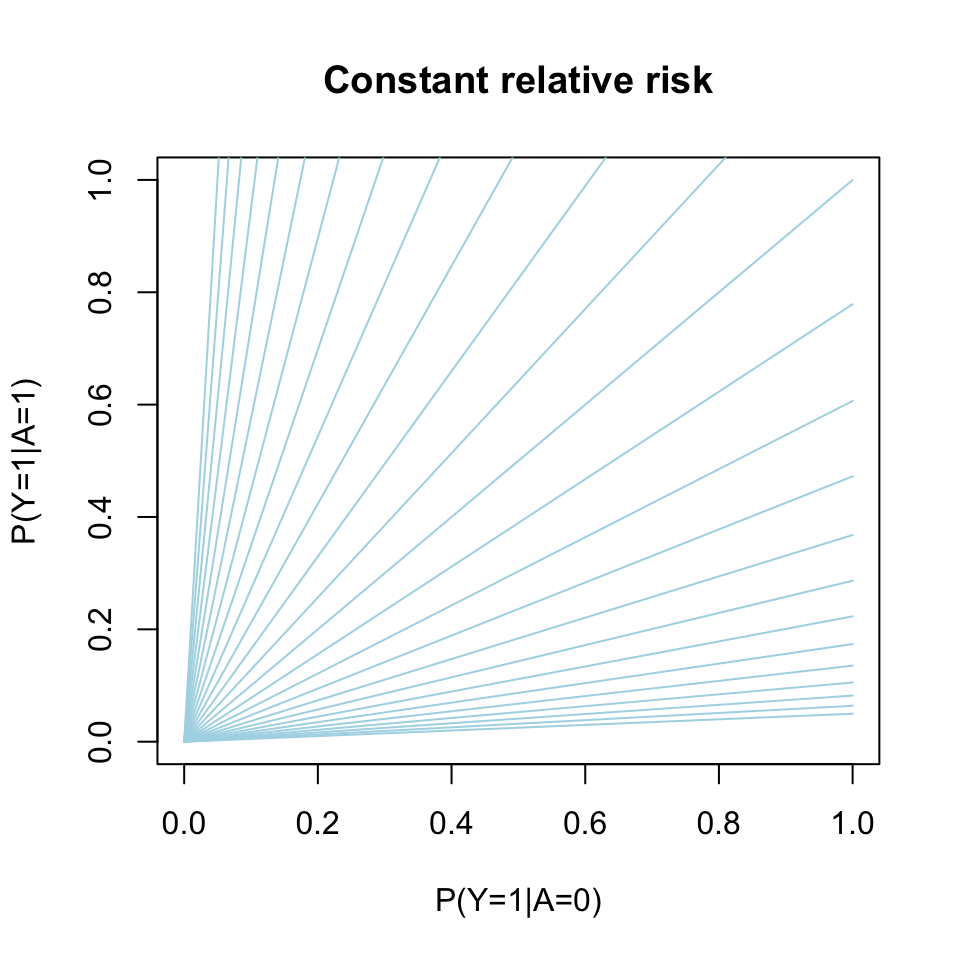# Introduction

Let $$Y$$ be a binary response, $$A$$ a binary exposure, and $$V$$ a vector of covariates.DAG for the statistical model with the dashed edge representing a potential interaction between exposure $$A$$ and covariates $$V$$.

In a common setting, the main interest lies in quantifying the treatment effect, $$\nu$$, of $$A$$ on $$Y$$ adjusting for the set of covariates, and often a standard approach is to use a Generalized Linear Model (GLM):

$g\{ E(Y\mid A,V) \} = A\nu^tW + \underset{\mathrm{nuisance}}{\mu^tZ}$

with link function $$g$$, and $$W = w(V)$$, $$Z= v(V)$$ known vector functions of $$V$$.

The canonical link (logit) leads to nice computational properties (logistic regression) and parameters with an odds-ratio interpretation. But ORs are not collapsible even under randomization. For example

$E(Y\mid X) = E[ E(Y\mid X,Z) \mid X ] = E[\operatorname{expit}( \mu + \alpha X + \beta Z ) \mid X] \neq \operatorname{expit}[\mu + \alpha X + \beta E(Z\mid X)],$

When marginalizing we leave the class of logistic regression. This non-collapsibility makes it hard to interpret odds-ratios and to compare results from different studies

Relative risks (and risk differences) are collapsible and generally considered easier to interpret than odds-ratios. Richardson et al (JASA, 2017) proposed a regression model for a binary exposures which solves the computational problems and need for parameter contraints that are associated with using for example binomial regression with a log-link function (or identify link for the risk difference) to obtain such parameter estimates. In the following we consider the relative risk as the target parameter

$\mathrm{RR}(v) = \frac{P(Y=1\mid A=1, V=v)}{P(Y=1\mid A=0, V=v)}.$

Let $$p_a(V) = P(Y \mid A=a, V), a\in\{0,1\}$$, the idea is then to posit a linear model for $\theta(v) = \log \big(RR(v)\big)$, i.e., $\log \big(RR(v)\big) = \alpha^Tv,$

and a nuisance model for the odds-product $\phi(v) = \log\left(\frac{p_{0}(v)p_{1}(v)}{(1-p_{0}(v))(1-p_{1}(v))}\right)$

noting that these two parameters are variation independent as illustrated by the below L’Abbé plot.

  p0 <- seq(0,1,length.out=100)
p1 <- function(p0,op) 1/(1+(op*(1-p0)/p0)^-1)
plot(0, type="n", xlim=c(0,1), ylim=c(0,1),
xlab="P(Y=1|A=0)", ylab="P(Y=1|A=1)", main="Constant odds product")
for (op in exp(seq(-6,6,by=.25))) lines(p0,p1(p0,op), col="lightblue")p0 <- seq(0,1,length.out=100)
p1 <- function(p0,rr) rr*p0
plot(0, type="n", xlim=c(0,1), ylim=c(0,1),
xlab="P(Y=1|A=0)", ylab="P(Y=1|A=1)", main="Constant relative risk")
for (rr in exp(seq(-3,3,by=.25))) lines(p0,p1(p0,rr), col="lightblue")Similarly, a model can be constructed for the risk-difference on the following scale

$\theta(v) = \operatorname{arctanh} \big(RD(v)\big).$

# Simulation

First the targeted package is loaded

library(targeted)

This automatically imports lava (CRAN) which we can use to simulate from the Relative-Risk Odds-Product (RR-OP) model.

m <- lvm(a ~ x,
lp.target ~ 1,
lp.nuisance ~ x+z)
m <- binomial.rr(m, response="y", exposure="a", target.model="lp.target", nuisance.model="lp.nuisance")

The lvm call first defines the linear predictor for the exposure to be of the form

$\mathrm{LP}_A := \mu_A + \alpha X$

and the linear predictors for the /target parameter/ (relative risk) and the /nuisance parameter/ (odds product) to be of the form

$\mathrm{LP}_{RR} := \mu_{RR},$

$\mathrm{LP}_{OP} := \mu_{OP} + \beta_x X + \beta_z Z.$

The covariates are by default assumed to be independent and standard normal $$X, Z\sim\mathcal{N}(0,1)$$, but their distribution can easily be altered using the lava::distribution method.

The binomial.rr function

args(binomial.rr)
#> function (x, response, exposure, target.model, nuisance.model,
#>     exposure.model = binomial.lvm(), ...)
#> NULL

then defines the link functions, i.e.,

$\operatorname{logit}(E[A\mid X,Z]) = \mu_A + \alpha X,$

$\operatorname{log}(E[Y\mid X,Z, A=1]/E[Y\mid X, A=0]) = \mu_{RR},$

$\operatorname{log}\{p_1(X,Z)p_0(X,Z)/[(1-p_1(X,Z))(1-p_0(X,Z))]\} = \mu_{OP}+\beta_x X + \beta_z Z$

with $$p_a(X,Z)=E(Y\mid A=a,X,Z)$$.

The risk-difference model with the RD parameter modeled on the $$\operatorname{arctanh}$$ scale can be defined similarly using the binomial.rd method

args(binomial.rd)
#> function (x, response, exposure, target.model, nuisance.model,
#>     exposure.model = binomial.lvm(), ...)
#> NULL

We can inspect the parameter names of the modeled

coef(m)
#>              m1              m2              m3              p1              p2
#>             "a"     "lp.target"   "lp.nuisance"           "a~x" "lp.nuisance~x"
#>              p3              p4
#> "lp.nuisance~z"          "a~~a"

Here the intercepts of the model are simply given the same name as the variables, such that $$\mu_A$$ becomes a, and the other regression coefficients are labeled using the “~”-formula notation, e.g., $$\alpha$$ becomes a~x.

Intercepts are by default set to zero and regression parameters set to one in the simulation. Hence to simulate from the model with $$(mu_A, \mu_{RR}, \mu_{OP}, \alpha, \beta_x, \beta_z)^T = (-1,1,-2,2,1,1)^T$$, we define the parameter vector p given by

p <- c('a'=-1, 'lp.target'=1, 'lp.nuisance'=-1, 'a~x'=2)

and then simulate from the model using the sim method

d <- sim(m, 1e4, p=p, seed=1)

#>   a          x lp.target lp.nuisance          z y
#> 1 0 -0.6264538         1  -2.4307854 -0.8043316 0
#> 2 0  0.1836433         1  -1.8728823 -1.0565257 0
#> 3 0 -0.8356286         1  -2.8710244 -1.0353958 0
#> 4 1  1.5952808         1  -0.5902796 -1.1855604 1
#> 5 0  0.3295078         1  -1.1709317 -0.5004395 1
#> 6 0 -0.8204684         1  -2.3454571 -0.5249887 0

Notice, that in this simulated data the target parameter $$\mu_{RR}$$ has been set to lp.target = 1.

# Estimation

## MLE

We start by fitting the model using the maximum likelihood estimator.

args(riskreg_mle)
#> function (y, a, x1, x2 = x1, weights = rep(1, length(y)), std.err = TRUE,
#>     type = "rr", start = NULL, control = list(), ...)
#> NULL

The riskreg_mle function takes vectors/matrices as input arguments with the response y, exposure a, target parameter design matrix x1 (i.e., the matrix $$W$$ at the start of this text), and the nuisance model design matrix x2 (odds product).

We first consider the case of a correctly specified model, hence we do not consider any interactions with the exposure for the odds product and simply let x1 be a vector of ones, whereas the design matrix for the log-odds-product depends on both $$X$$ and $$Z$$

x1 <- model.matrix(~1, d)
x2 <- model.matrix(~x+z, d)

fit1 <- with(d, riskreg_mle(y, a, x1, x2, type="rr"))
fit1
#>                          Estimate Std.Err    2.5%   97.5%    P-value
#> (Intercept)                0.9512 0.03319  0.8862  1.0163 1.204e-180
#> odds-product:(Intercept)  -1.0610 0.05199 -1.1629 -0.9591  1.377e-92
#> odds-product:x             1.0330 0.05944  0.9165  1.1495  1.230e-67
#> odds-product:z             1.0421 0.05285  0.9386  1.1457  1.523e-86

The parameters are presented in the same order as the columns of x1and x2, hence the target parameter estimate is in the first row

estimate(fit1, keep=1)
#>             Estimate Std.Err   2.5% 97.5%    P-value
#> (Intercept)   0.9512 0.03336 0.8858 1.017 7.159e-179

## DRE

We next fit the model using a double robust estimator (DRE) which introduces a model for the exposure $$E(A=1\mid V)$$ (propensity model). The double-robustness stems from the fact that the this estimator remains consistent in the union model where either the odds-product model or the propensity model is correctly specified. With both models correctly specified the estimator is efficient.

with(d, riskreg_fit(y, a, target=x1, nuisance=x2, propensity=x2, type="rr"))
#>             Estimate Std.Err   2.5% 97.5%    P-value
#> (Intercept)   0.9372  0.0339 0.8708 1.004 3.004e-168

The usual /formula/-syntax can be applied using the riskreg function. Here we illustrate the double-robustness by using a wrong propensity model but a correct nuisance paramter (odds-product) model:

  riskreg(y~a, nuisance=~x+z, propensity=~z, data=d, type="rr")
#>             Estimate Std.Err   2.5% 97.5%    P-value
#> (Intercept)   0.9511 0.03333 0.8857 1.016 4.547e-179

Or vice-versa

  riskreg(y~a, nuisance=~z, propensity=~x+z, data=d, type="rr")
#>             Estimate Std.Err   2.5% 97.5%    P-value
#> (Intercept)   0.9404 0.03727 0.8673 1.013 1.736e-140

whereas the MLE in this case yields a biased estimate of the relative risk:

  fit2 <- with(d, riskreg_mle(y, a, x1=model.matrix(~1,d), x2=model.matrix(~z, d)))
estimate(fit2, keep=1)
#>             Estimate Std.Err  2.5% 97.5% P-value
#> (Intercept)    1.243 0.02778 1.189 1.298       0

## Interactions

The more general model where $\log RR(V) = A \alpha^TV$ for a subset $$V$$ of the covariates can be estimated using the target argument:

fit <- riskreg(y~a, target=~x, nuisance=~x+z, data=d)
fit
#>             Estimate Std.Err    2.5%   97.5%    P-value
#> (Intercept)  0.93479 0.03528  0.8656 1.00393 9.881e-155
#> x           -0.02035 0.05259 -0.1234 0.08273  6.988e-01

As expected we do not see any evidence of an effect of $$X$$ on the relative risk with the 95% confidence limits clearly overlapping zero.

Note, that when the propensity argument is omitted as above, the same design matrix is used for both the odds-product model and the propensity model.

## Risk-difference

The syntax for fitting the risk-difference model is similar. To illustrate this we simulate some new data from this model

m2 <- binomial.rd(m, response="y", exposure="a", target.model="lp.target", nuisance.model="lp.nuisance")
d2 <- sim(m2, 1e4, p=p)

And we can then fit the DRE with the syntax

riskreg(y~a, nuisance=~x+z, data=d2, type="rd")
#>             Estimate Std.Err   2.5% 97.5% P-value
#> (Intercept)    1.011 0.02098 0.9704 1.053       0

## Influence-function

The DRE is a regular and asymptotic linear (RAL) estimator, hence $\sqrt{n}(\widehat{\alpha}_{\mathrm{DRE}} - \alpha) = \frac{1}{\sqrt{n}}\sum_{i=1}^{n} \phi_{\mathrm{eff}}(Z_{i}) + o_{p}(1)$ where $$Z_i = (Y_i, A_i, V_i), i=1,\ldots,n$$ are the i.i.d. observations and $$\phi_{\mathrm{eff}}$$ is the influence function.

The influence function can be extracted using the iid method

head(iid(fit))
#>        (Intercept)             x
#> [1,]  6.283230e-05 -9.100844e-05
#> [2,]  1.454404e-04  2.519557e-06
#> [3,]  3.801776e-05 -6.489639e-05
#> [4,]  5.305096e-06  8.331332e-05
#> [5,] -7.976368e-04 -2.798109e-04
#> [6,]  5.316760e-05 -8.983380e-05

# SessionInfo

sessionInfo()
#> R version 4.2.1 (2022-06-23)
#> Platform: aarch64-apple-darwin21.6.0 (64-bit)
#> Running under: macOS Monterey 12.6
#>
#> Matrix products: default
#> BLAS:   /Users/Klaus.Holst/.asdf/installs/R/4.2.1/lib/R/lib/libRblas.dylib
#> LAPACK: /Users/Klaus.Holst/.asdf/installs/R/4.2.1/lib/R/lib/libRlapack.dylib
#>
#> locale:
#>  en_US.UTF-8/en_US.UTF-8/en_US.UTF-8/C/en_US.UTF-8/en_US.UTF-8
#>
#> attached base packages:
#>  stats     graphics  grDevices utils     datasets  methods   base
#>
#> other attached packages:
#>  targeted_0.3 lava_1.7.0
#>
#> loaded via a namespace (and not attached):
#>   Rcpp_1.0.9           highr_0.9            bslib_0.4.0
#>   compiler_4.2.1       formatR_1.12         jquerylib_0.1.4
#>   futile.logger_1.4.3  mets_1.3.1           futile.options_1.0.1
#>  tools_4.2.1          digest_0.6.30        jsonlite_1.8.3
#>  evaluate_0.17        lattice_0.20-45      rlang_1.0.6
#>  Matrix_1.5-1         cli_3.4.1            yaml_2.3.6
#>  parallel_4.2.1       mvtnorm_1.1-3        xfun_0.34
#>  fastmap_1.1.0        stringr_1.4.1        knitr_1.40
#>  sass_0.4.2           globals_0.16.1       grid_4.2.1
#>  data.table_1.14.4    listenv_0.8.0        R6_2.5.1
#>  timereg_2.0.2        future.apply_1.9.1   parallelly_1.32.1
#>  survival_3.3-1       rmarkdown_2.17       lambda.r_1.2.4
#>  magrittr_2.0.3       codetools_0.2-18     htmltools_0.5.3
#>  splines_4.2.1        future_1.28.0        numDeriv_2016.8-1.1
#>  optimx_2022-4.30     stringi_1.7.8        cachem_1.0.6